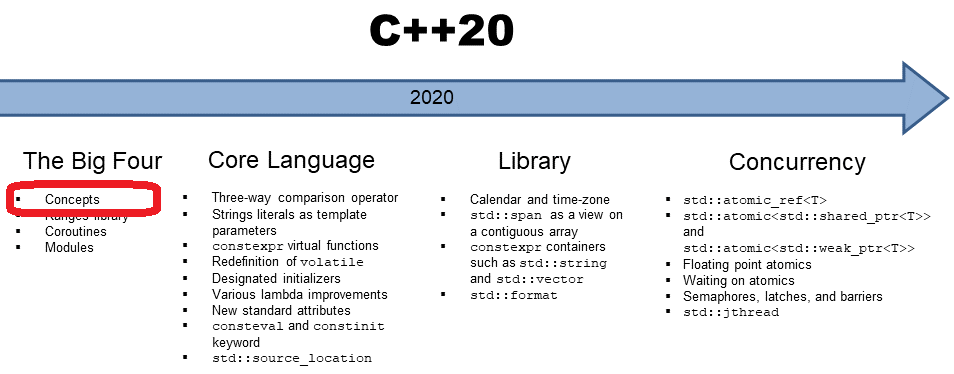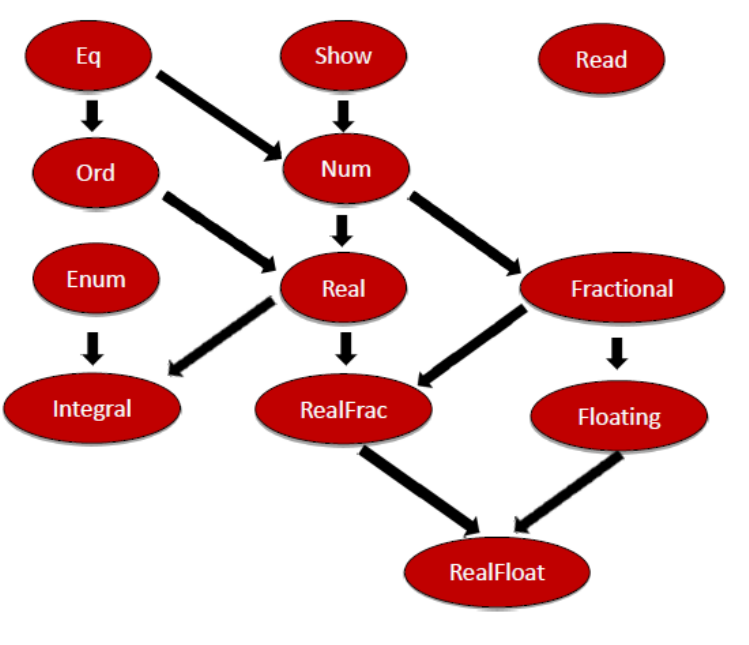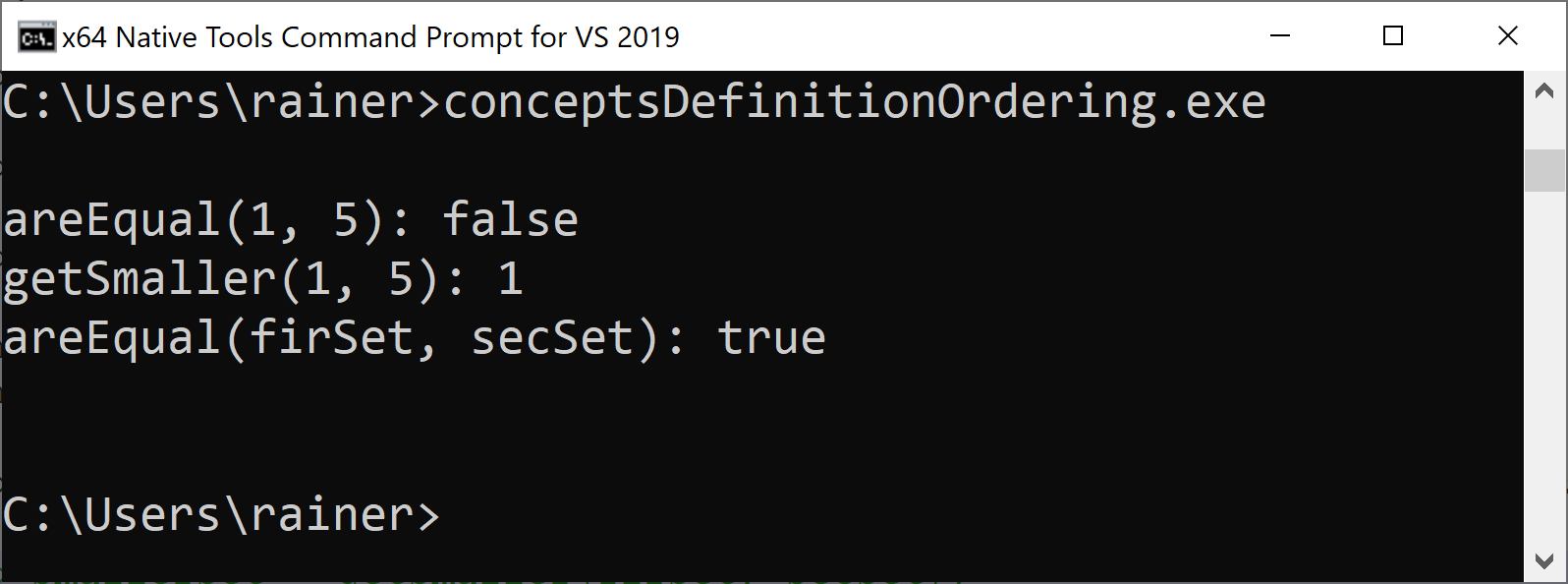# C++20: Define the Concepts Equal and Ordering

In my last post, I defined the concept Equal. Now, I go one step further and use the concept Equal to define the concept Ordering.Here is a short reminder of where I ended with my last post. I defined the concept of Equal and a function areEqual to use it.

```template<typename T>
concept Equal =
requires(T a, T b) {
{ a == b } -> bool;
{ a != b } -> bool;
};

bool areEqual(Equal auto fir, Equal auto sec) {
return fir == sec;
}
```

### My Wrong Usage of the Concept Equal

I used the concept of Equal in my last post in the wrong way. The concept Equal requires that a and b have the same type but the function areEqual allows fir and sec to be different types that both support the concept Equal. Using a constrained template parameter instead of placeholder syntax solves the issue:

##Modernes C++ Mentoring

Be part of my mentoring programs:

• "Fundamentals for C++ Professionals" (open)
• "Design Patterns and Architectural Patterns with C++" (open)
• "C++20: Get the Details" (reopens December 2023)
• Do you want to stay informed about my mentoring programs: Subscribe via E-Mail.

```template <Equal T>
bool areEqual(T fir, T sec) {
fir == sec;
}
```

Now, fir and sec must have the same type.

Thanks a lot to Corentin Jabot for pointing out this inconsistency.

Additionally, the concept Equal should not check if the equal and unequal operator returns a bool but something implicitly or explicitly convertible to a bool. Here we are.

```template<typename T>
concept Equal =
requires(T a, T b) {
{ a == b } -> std::convertible_to<bool>;
{ a != b } -> std::convertible_to<bool>;
};
```

I have to add. is a concept and requires the header <concepts>.

```template <class From, class To>
concept convertible_to =
std::is_convertible_v<From, To> &&
requires(From (&f)()) {
static_cast<To>(f());
};
```

The C++ 20 standard has already defined two concepts for equality comparing:

• std::equality_comparable: corresponds to my concept Equal
• std::equality_comparable_with: allows the comparison of values of different type; e.g.: 1.0 == 1.0f

## The Challenge

I ended my last post by presenting a part of the type class hierarchy of Haskell.The class hierarchy shows that the type class Ord is a refinement of the type class Eq. This can elegantly be expressed in Haskell.

```class Eq a where
(==) :: a -> a -> Bool
(/=) :: a -> a -> Bool

class Eq a => Ord a where
compare :: a -> a -> Ordering
(<) :: a -> a -> Bool
(<=) :: a -> a -> Bool
(>) :: a -> a -> Bool
(>=) :: a -> a -> Bool
max :: a -> a -> a
```

Here is my challenge. Can I express such as relationship quite elegantly with concepts in C++20? For simplicity reasons, I ignore the functions compare and max of Haskell’s type class. Of course, I can.

## The Concept Ordering

Thanks to requires-expression, the definition of the concept Ordering looks quite similar to the definition of the type class Equal.

```template <typename T>
concept Ordering =
Equal<T> &&
requires(T a, T b) {
{ a <= b } -> std::convertible_to<bool>;
{ a < b } -> std::convertible_to<bool>;
{ a > b } -> std::convertible_to<bool>;
{ a >= b } -> std::convertible_to<bool>;
};
```

Okay, let me try it out.

```// conceptsDefinitionOrdering.cpp

#include <concepts>
#include <iostream>
#include <unordered_set>

template<typename T>
concept Equal =
requires(T a, T b) {
{ a == b } -> std::convertible_to<bool>;
{ a != b } -> std::convertible_to<bool>;
};

template <typename T>
concept Ordering =
Equal<T> &&
requires(T a, T b) {
{ a <= b } -> std::convertible_to<bool>;
{ a < b } -> std::convertible_to<bool>;
{ a > b } -> std::convertible_to<bool>;
{ a >= b } -> std::convertible_to<bool>;
};

template <Equal T>
bool areEqual(T a, T b) {
return a == b;
}

template <Ordering T>
T getSmaller(T a, T b) {
return (a < b) ? a : b;
}

int main() {

std::cout << std::boolalpha << std::endl;

std::cout << "areEqual(1, 5): " << areEqual(1, 5) << std::endl;

std::cout << "getSmaller(1, 5): " << getSmaller(1, 5) << std::endl;

std::unordered_set<int> firSet{1, 2, 3, 4, 5};
std::unordered_set<int> secSet{5, 4, 3, 2, 1};

std::cout << "areEqual(firSet, secSet): " << areEqual(firSet, secSet) << std::endl;

// auto smallerSet = getSmaller(firSet, secSet);

std::cout << std::endl;

}
```

The function getSmaller requires that both arguments a and b support the concept Ordering, and both have the same type. This requirement holds for numbers 1 and 5.Of course, a std::unordered_set does not support order. The actual MSVC compiler is very specific when I try to compile the line auto smaller = getSmaller(firSet, secSet) with the flag /std:c++latest.By the way. The error message is obvious: the associated constraints are not satisfied.

Of course, the concept Ordering is already part of the C++20 standard.

• std::three_way_comparable: corresponds to my concept Ordering
• std::three_way_comparable_with: allows the comparison of values of different type; e.g.: 1.0 < 1.0f

Maybe, you are irritated by the term three-way. With C++20, we get the three-way comparison operator, the spaceship operator. <=>. Here is the first overview: C++20: The Core Language. I will write about the three-way comparison operator in a future post.

### Compiler Support

I learn new stuff by trying it out. Maybe, you don’t have an actual MSVC available. In this case, use the current GCC (trunk) on the Compiler Explorer. GCC supports the C++20 syntax for concepts. Here is the conceptsDefinitionOrdering.cpp for further experiments: https://godbolt.org/z/uyVFX8.

## What’s next?

When you want to define a concrete type that works well in the C++ ecosystem, you should define a type that “behaves link an int“.  Such a concrete type could be copied and the result of the copy operation is independent of the original one and has the same value.  Formally, your concrete type should be regular. In the next post, I will define the concepts Regular and SemiRegular.

Thanks a lot to my Patreon Supporters: Matt Braun, Roman Postanciuc, Tobias Zindl, G Prvulovic, Reinhold Dröge, Abernitzke, Frank Grimm, Sakib, Broeserl, António Pina, Sergey Agafyin, Андрей Бурмистров, Jake, GS, Lawton Shoemake, Jozo Leko, John Breland, Venkat Nandam, Jose Francisco, Douglas Tinkham, Kuchlong Kuchlong, Robert Blanch, Truels Wissneth, Kris Kafka, Mario Luoni, Friedrich Huber, lennonli, Pramod Tikare Muralidhara, Peter Ware, Daniel Hufschläger, Alessandro Pezzato, Bob Perry, Satish Vangipuram, Andi Ireland, Richard Ohnemus, Michael Dunsky, Leo Goodstadt, John Wiederhirn, Yacob Cohen-Arazi, Florian Tischler, Robin Furness, Michael Young, Holger Detering, Bernd Mühlhaus, Matthieu Bolt, Stephen Kelley, Kyle Dean, Tusar Palauri, Dmitry Farberov, Juan Dent, George Liao, Daniel Ceperley, Jon T Hess, Stephen Totten, Wolfgang Fütterer, Matthias Grün, Phillip Diekmann, Ben Atakora, Ann Shatoff, Rob North, Bhavith C Achar, and Marco Parri Empoli.

Thanks, in particular, to Jon Hess, Lakshman, Christian Wittenhorst, Sherhy Pyton, Dendi Suhubdy, Sudhakar Belagurusamy, Richard Sargeant, Rusty Fleming, John Nebel, Mipko, Alicja Kaminska, Slavko Radman, and David Poole.

 My special thanks to EmbarcaderoMy special thanks to PVS-StudioMy special thanks to Tipi.buildMy special thanks to Take Up Code## Seminars

I’m happy to give online seminars or face-to-face seminars worldwide. Please call me if you have any questions.

### Standard Seminars (English/German)

Here is a compilation of my standard seminars. These seminars are only meant to give you a first orientation.

• C++ – The Core Language
• C++ – The Standard Library
• C++ – Compact
• C++11 and C++14
• Concurrency with Modern C++
• Design Pattern and Architectural Pattern with C++
• Embedded Programming with Modern C++
• Generic Programming (Templates) with C++

#### New

• Clean Code with Modern C++
• C++20

### Contact Me

Modernes C++ Mentoring,Tags:
0 replies